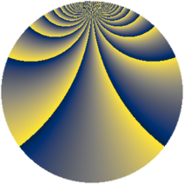Properties

 Label 1089.3.baLevel $1089$ Weight $3$ Character orbit 1089.ba Rep. character $\chi_{1089}(19,\cdot)$ Character field $\Q(\zeta_{110})$ Dimension $4360$ Sturm bound $396$

Related objects

Defining parameters

 Level: $$N$$ $$=$$ $$1089 = 3^{2} \cdot 11^{2}$$ Weight: $$k$$ $$=$$ $$3$$ Character orbit: $$[\chi]$$ $$=$$ 1089.ba (of order $$110$$ and degree $$40$$) Character conductor: $$\operatorname{cond}(\chi)$$ $$=$$ $$121$$ Character field: $$\Q(\zeta_{110})$$ Sturm bound: $$396$$

Dimensions

The following table gives the dimensions of various subspaces of $$M_{3}(1089, [\chi])$$.

Total New Old
Modular forms 10720 4440 6280
Cusp forms 10400 4360 6040
Eisenstein series 320 80 240

Trace form

 $$4360 q + 39 q^{2} - 251 q^{4} + 39 q^{5} - 54 q^{7} + 19 q^{8} + O(q^{10})$$ $$4360 q + 39 q^{2} - 251 q^{4} + 39 q^{5} - 54 q^{7} + 19 q^{8} + 44 q^{10} + 90 q^{11} - 35 q^{13} + 72 q^{14} + 485 q^{16} + 34 q^{17} - 69 q^{19} + 86 q^{20} - 53 q^{22} - 72 q^{23} + 520 q^{25} + 100 q^{26} + 116 q^{28} + 164 q^{29} - 107 q^{31} + 44 q^{32} + 42 q^{34} - 196 q^{35} - 160 q^{37} - 496 q^{38} - 475 q^{40} - 156 q^{41} - 44 q^{43} - 281 q^{44} + 246 q^{46} - 70 q^{47} - 873 q^{49} - 815 q^{50} + 240 q^{52} + 832 q^{53} - 88 q^{55} + 575 q^{56} + 399 q^{58} + 174 q^{59} - 174 q^{61} - 321 q^{62} - 1567 q^{64} + 275 q^{65} - 203 q^{67} - 136 q^{68} - 108 q^{70} - 433 q^{71} + 462 q^{73} - 956 q^{74} - 1265 q^{76} + 246 q^{77} + 658 q^{79} + 873 q^{80} + 129 q^{82} + 79 q^{83} + 130 q^{85} + 893 q^{86} - 831 q^{88} + 383 q^{89} - 795 q^{91} + 1730 q^{92} - 855 q^{94} + 110 q^{95} + 93 q^{97} - 1628 q^{98} + O(q^{100})$$

Decomposition of $$S_{3}^{\mathrm{new}}(1089, [\chi])$$ into newform subspaces

The newforms in this space have not yet been added to the LMFDB.

Decomposition of $$S_{3}^{\mathrm{old}}(1089, [\chi])$$ into lower level spaces

$$S_{3}^{\mathrm{old}}(1089, [\chi]) \cong$$ $$S_{3}^{\mathrm{new}}(121, [\chi])$$$$^{\oplus 3}$$$$\oplus$$$$S_{3}^{\mathrm{new}}(363, [\chi])$$$$^{\oplus 2}$$